# Exterior Angle Theorem

Go back to  'Triangles'

Consider the following figure, in which the side BC of a triangle ABC has been extended.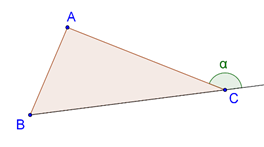The angle marked α is an example of an exterior angle for the triangle ABC. The following figure shows two more exterior angles for the same triangle: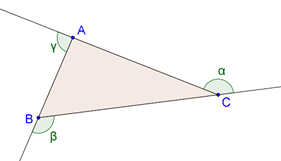A very important consequence of the angle sum property of triangles is the exterior angle theorem: an exterior angle in any triangle is equal to the sum of the opposite interior angles. For example, in the following figure, α must be equal to x + y: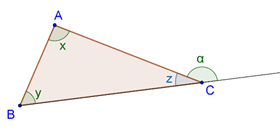Proof: The proof of this theorem is straightforward. Referring to the figure above, we have:

x + y + z = 1800 (angle sum property)

$$\alpha {\rm{ }} + {\rm{ }}z{\rm{ }} = {\rm{ }}{180^0}$$ (linear pair)

Thus,

$$\alpha {\rm{ }} = {\rm{ x + y}}$$

Example 1: Consider the following figure: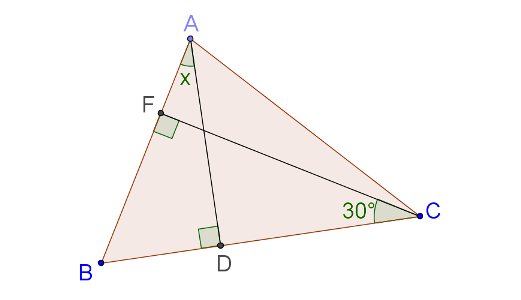What is the measure of $$\angle {\rm{x}}$$?

Solution: We apply the angle sum property to $$\Delta {\rm{ABD}}$$ and $$\Delta {\rm{CBF}}$$:

$$\angle {\rm{x }} + \angle {\rm{B }} + \angle {\rm{ADB }} = {\rm{ }}{180^0}$$

∠BCF + ∠B + ∠CFB = 1800

We note that $$\angle {\rm{ADB }} = \angle {\rm{CFB }} = {\rm{ }}{90^0}$$. Thus,

$$\angle {\rm{x }} + \angle {\rm{B }} + \angle {\rm{ADB }} = \angle {\rm{BCF }} + \angle {\rm{B }} + \angle {\rm{CFB}}$$

è$$\angle {\rm{x }} = \angle {\rm{BCF}}$$

è$$\angle {\rm{x }} = {\rm{ }}{30^0}$$

Example 2: Consider the following figure, in which BF and CF are bisectors of the external angles at B and C: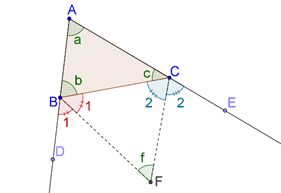Express $$\angle {\rm{f}}$$ in terms of$$\angle {\rm{a}}$$.

Solution: We have:

$$\angle {\rm{b }} + {\rm{ }}2\angle 1{\rm{ }} = {\rm{ }}{180^0}$$

è$$\angle {\rm{b }} = {\rm{ }}{180^0}--{\rm{ }}2\angle 1$$

Similarly, we have:

$$\angle {\rm{c }} = {\rm{ }}{180^0}--{\rm{ }}2\angle 2$$

Now, we apply the angle sum property to ΔABC:

$$\angle {\rm{a }} + \angle {\rm{b }} + \angle {\rm{c }} = {\rm{ }}{180^0}$$

è$$\angle {\rm{a }} + {\rm{ }}({180^0}--{\rm{ }}2\angle 1){\rm{ }} + {\rm{ }}({180^0}--{\rm{ }}2\angle 2){\rm{ }} = {\rm{ }}{180^0}$$

è$$2(\angle 1{\rm{ }} + \angle 2){\rm{ }} = {\rm{ }}{180^0} + \angle {\rm{a}}$$

è$$\angle 1{\rm{ }} + \angle 2{\rm{ }} = {\rm{ }}{90^0} + \frac{1}{2}\angle {\rm{a}}$$

Now, we apply the angle sum property to $$\Delta {\rm{BCF}}$$:

$$\angle 1{\rm{ }} + \angle 2{\rm{ }} + \angle {\rm{f }} = {\rm{ }}{180^0}$$

è$$({90^0} + \frac{1}{2}\angle {\rm{a}}){\rm{ }} + \angle {\rm{f }} = {\rm{ }}{180^0}$$

è$$\angle {\rm{f }} = {\rm{ }}{90^0} - \frac{1}{2}\angle {\rm{a}}$$

This completes our solution.

Example 3: Consider the following figure, in which side BA of $$\Delta {\rm{ABC}}$$ is extended up to D, and ray DE through D is parallel to BC. What is the value of $$\angle {\rm{a}}$$?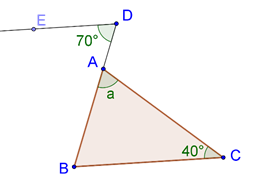Solution: Since $${\rm{DE}}\parallel {\rm{BC}}$$, we have:

$$\angle {\rm{B }} = \angle {\rm{D}}$$ (alternate interior angles)

è$$\angle {\rm{B }} = {\rm{ }}{70^0}$$

Thus, $$\angle {\rm{B }} + \angle {\rm{C }} = {\rm{ }}{110^0}$$, and so by the angle sum property,$$\angle {\rm{a }} = {\rm{ }}{180^0}--{\rm{ }}{110^0} = {\rm{ }}{70^0}$$.

Example 4: Consider the following figure: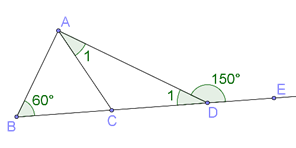What is the value of $$\angle {\rm{BAC}}$$ ?

Solution: We note that $$\angle 1$$ and $$\angle {\rm{ADE}}$$ form a linear pair, and so,$$\angle 1{\rm{ }} = {\rm{ }}{180^0}--{\rm{ }}{150^0} = {\rm{ }}{30^0}$$. Now, in $$\Delta {\rm{ABD}}$$, $$\angle {\rm{ADE}}$$ is an exterior angle, and by the exterior angle theorem, we have:

$$\angle {\rm{ADE }} = \angle {\rm{B }} + \angle {\rm{BAD}}$$

è$${150^0} = {\rm{ }}{60^0} + \angle {\rm{BAD}}$$

è$$\angle {\rm{BAD }} = {\rm{ }}{90^0}$$

Finally, we have:

$$\angle {\rm{BAC }} = \angle {\rm{BAD }} - \angle 1{\rm{ }} = {\rm{ }}{90^0}--\angle 1{\rm{ }} = {\rm{ }}{60^0}$$

Example 5: Consider a heptagon (a polygon with seven sides) as shown below: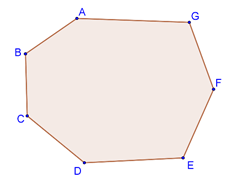What is the sum of the internal angles of this heptagon? Generalize your result to a polygon of n sides.

Solution: Take any point O in the interior of the heptagon, and join it to all the vertices: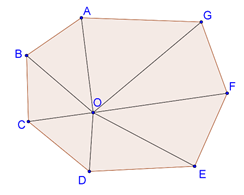Seven triangles are thus formed, and the sum of the angles of each triangle is 1800. Now, suppose that we sum the angles of all the seven triangles. Obviously, that sum should be 180 × 7 = 12600. But when we combine all the angles of all the seven triangles, there are seven angles which are formed at O (if we remove these angles, the remaining sum will be the sum of the interior angles of the heptagon):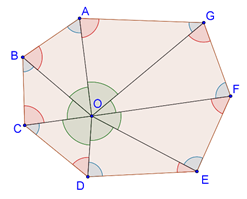The sum of these seven angles formed at O is 3600 (why?), so the sum of the remaining angles is 12600 – 3600, or 9000. This will be the sum of the interior angles of the heptagon.

In an n-sided polygon, if we similarly join an interior point O to all the vertices, n triangles will be formed, and the sum of the angles of each triangle will be 1800, so that total sum of all the angles will be 180n0. Out of this sum, there will be a contribution of 3600 by the angles which are formed at O. We need to discard that contribution because we want to find out the sum of only the interior angles. Thus, the net sum will be (180n – 360)0, or 180(n – 2)0.

Example 6: Consider the heptagon from the previous example once again. This time, we have to find the sum of the exterior angles, as shown in the figure: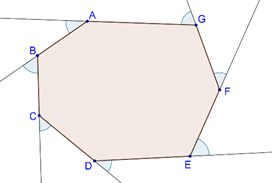Solution: There is a very good intuitive way to think about the solution to this problem. Suppose that you are standing at the point A, and you starting walking along the edges of the heptagon: A → B →C → D → E → F → G → A. At each vertex, your direction changes by a certain angle. However, once you reach back to point A, you end up facing in your original direction. Suppose that instead of walking along the edges, you had stood at one fixed point, only changing the direction in which you were looking. After one rotation, you would end up facing in your original direction once again. And through what angle will you have turned after one rotation? Obviously, 3600. Thus, the answer to this problem should also be 3600. In fact, in any polygon, the answer should stay the same (well, there is a case where things get a little trickier – concave polygons – but for now, we will not get into that). Let us prove our intuition rigorously.

Each exterior angle is simply 1800 minus an interior angle. For example, note in the figure above that the exterior angle at A is 1800 minus the interior angle at A. Thus, any exterior angle = 1800 – Corresponding interior angle:

$${\rm{Exterior}}\angle {\rm{A }} = {\rm{ }}{180^0}-{\rm{ Interior}}\angle {\rm{A}}$$

$${\rm{Exterior}}\angle {\rm{B }} = {\rm{ }}{180^0}-{\rm{ Interior}}\angle {\rm{B}}$$

$${\rm{Exterior}}\angle {\rm{C }} = {\rm{ }}{180^0}-{\rm{ Interior}}\angle {\rm{C}}$$

$${\rm{Exterior}}\angle {\rm{D }} = {\rm{ }}{180^0}-{\rm{ Interior}}\angle {\rm{D}}$$

$${\rm{Exterior}}\angle {\rm{E }} = {\rm{ }}{180^0}-{\rm{ Interior}}\angle {\rm{E}}$$

$${\rm{Exterior}}\angle {\rm{F }} = {\rm{ }}{180^0}-{\rm{ Interior}}\angle {\rm{F}}$$

$${\rm{Exterior}}\angle {\rm{G }} = {\rm{ }}{180^0}-{\rm{ Interior}}\angle {\rm{G}}$$

If we add all these relations, we have:

Exterior Sum = (180 × 7)0 – Interior Sum

è Exterior Sum = (180 × 7)0 – (180 × 5)0

è Exterior Sum = (180 × 2)0 = 3600

This same reasoning can easily be extended to a polygon with n sides:

Exterior Sum = (180 × n)0 – Interior Sum

è Exterior Sum = (180 × n)0 – (180 × (n – 2))0

è Exterior Sum = (180 × 2)0 = 3600

Geometry
grade 9 | Questions Set 1
Geometry
Geometry
grade 9 | Questions Set 2
Geometry